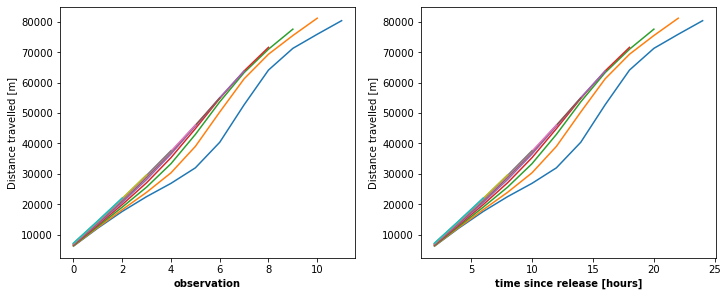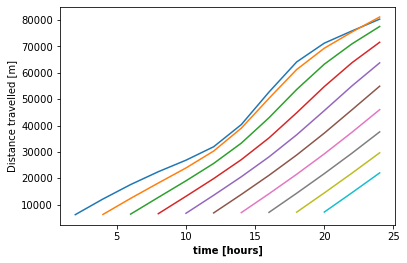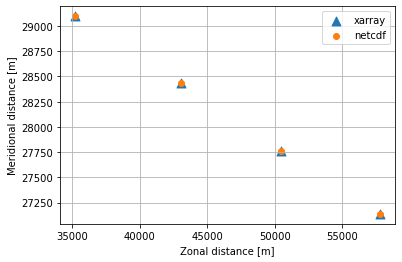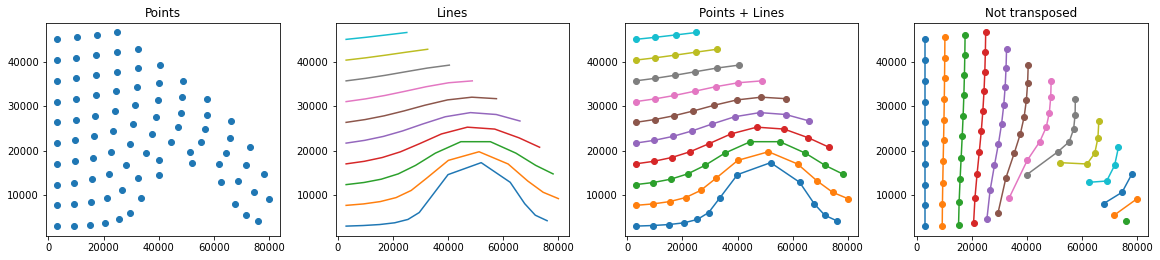# Tutorial on how to analyse Parcels output¶

This tutorial covers the format of the trajectory output exported by Parcels. Parcels does not include advanced analysis or plotting functionality, which users are suggested to write themselves to suit their research goals. Here we provide some starting points to explore the parcels output files yourself.

First we need to create some parcels output to analyse. We simulate a set of particles using the setup described in the Delay start tutorial.

In :
from parcels import FieldSet, ParticleSet, JITParticle
import numpy as np
from datetime import timedelta as delta

In :
fieldset = FieldSet.from_parcels("Peninsula_data/peninsula", allow_time_extrapolation=True)

npart = 10  # number of particles to be released
lon = 3e3 * np.ones(npart)
lat = np.linspace(3e3 , 45e3, npart, dtype=np.float32)
time = np.arange(0, npart) * delta(hours=2).total_seconds()  # release every particle two hours later

pset = ParticleSet(fieldset=fieldset, pclass=JITParticle, lon=lon, lat=lat, time=time)

output_file = pset.ParticleFile(name="Output.nc", outputdt=delta(hours=2))
output_file=output_file)
output_file.close()  # export the trajectory data to a netcdf file

INFO: Compiled JITParticleAdvectionRK4 ==> C:\Users\GEBRUI~1\AppData\Local\Temp\parcels-tmp\41e0d83670ae127d5c88fa24315b7a08_0.dll


### Using the netCDF4 package¶

The parcels.particlefile.ParticleFile class creates a netCDF file to store the particle trajectories. It uses the netCDF4 package, which is also suitable to open and read the files for analysis. The Dataset class opens a netCDF file in reading mode by default. Data can be accessed with the Dataset.variables dictionary which can return (masked) numpy arrays.

In :
import netCDF4

data_netcdf4 = netCDF4.Dataset('Output.nc')
print(data_netcdf4)

<class 'netCDF4._netCDF4.Dataset'>
root group (NETCDF4 data model, file format HDF5):
feature_type: trajectory
Conventions: CF-1.6/CF-1.7
ncei_template_version: NCEI_NetCDF_Trajectory_Template_v2.0
parcels_version: 2.2.1.dev2+ga8340d9b
parcels_mesh: flat
dimensions(sizes): obs(13), traj(10)
variables(dimensions): int32 trajectory(traj, obs), float64 time(traj, obs), float32 lat(traj, obs), float32 lon(traj, obs), float32 z(traj, obs)
groups:

In :
trajectory_netcdf4 = data_netcdf4.variables['trajectory'][:]
time_netcdf4 = data_netcdf4.variables['time'][:]
lon_netcdf4 = data_netcdf4.variables['lon'][:]
lat_netcdf4 = data_netcdf4.variables['lat'][:]
print(trajectory_netcdf4)

[[0 0 0 0 0 0 0 0 0 0 0 0 0]
[1 1 1 1 1 1 1 1 1 1 1 1 --]
[2 2 2 2 2 2 2 2 2 2 2 -- --]
[3 3 3 3 3 3 3 3 3 3 -- -- --]
[4 4 4 4 4 4 4 4 4 -- -- -- --]
[5 5 5 5 5 5 5 5 -- -- -- -- --]
[6 6 6 6 6 6 6 -- -- -- -- -- --]
[7 7 7 7 7 7 -- -- -- -- -- -- --]
[8 8 8 8 8 -- -- -- -- -- -- -- --]
[9 9 9 9 -- -- -- -- -- -- -- -- --]]


### Using the xarray package¶

An often-used alternative to netCDF4, which also comes with the parcels installation, is xarray. Its labelled arrays allow for intuitive and accessible handling of data stored in the netCDF format.

In :
import xarray as xr

data_xarray = xr.open_dataset('Output.nc')
print(data_xarray)

<xarray.Dataset>
Dimensions:     (obs: 13, traj: 10)
Dimensions without coordinates: obs, traj
Data variables:
trajectory  (traj, obs) float64 ...
time        (traj, obs) timedelta64[ns] ...
lat         (traj, obs) float32 ...
lon         (traj, obs) float32 ...
z           (traj, obs) float32 ...
Attributes:
feature_type:           trajectory
Conventions:            CF-1.6/CF-1.7
ncei_template_version:  NCEI_NetCDF_Trajectory_Template_v2.0
parcels_version:        2.2.1.dev2+ga8340d9b
parcels_mesh:           flat

In :
print(data_xarray['trajectory'])

<xarray.DataArray 'trajectory' (traj: 10, obs: 13)>
array([[ 0.,  0.,  0.,  0.,  0.,  0.,  0.,  0.,  0.,  0.,  0.,  0.,  0.],
[ 1.,  1.,  1.,  1.,  1.,  1.,  1.,  1.,  1.,  1.,  1.,  1., nan],
[ 2.,  2.,  2.,  2.,  2.,  2.,  2.,  2.,  2.,  2.,  2., nan, nan],
[ 3.,  3.,  3.,  3.,  3.,  3.,  3.,  3.,  3.,  3., nan, nan, nan],
[ 4.,  4.,  4.,  4.,  4.,  4.,  4.,  4.,  4., nan, nan, nan, nan],
[ 5.,  5.,  5.,  5.,  5.,  5.,  5.,  5., nan, nan, nan, nan, nan],
[ 6.,  6.,  6.,  6.,  6.,  6.,  6., nan, nan, nan, nan, nan, nan],
[ 7.,  7.,  7.,  7.,  7.,  7., nan, nan, nan, nan, nan, nan, nan],
[ 8.,  8.,  8.,  8.,  8., nan, nan, nan, nan, nan, nan, nan, nan],
[ 9.,  9.,  9.,  9., nan, nan, nan, nan, nan, nan, nan, nan, nan]])
Dimensions without coordinates: traj, obs
Attributes:
long_name:  Unique identifier for each particle
cf_role:    trajectory_id


## Trajectory data structure¶

The data in the netCDF file are organised according to the CF-conventions implemented with the NCEI trajectory template. The data is stored in a two-dimensional array with the dimensions traj and obs. Each particle trajectory is essentially stored as a time series where the coordinate data (lon, lat, time) are a function of the observation (obs).

The output dataset used here contains 10 particles and 13 observations. Not every particle has 13 observations however; since we released particles at different times some particle trajectories are shorter than others.

In :
np.set_printoptions(linewidth=160)
ns_per_hour = np.timedelta64(1, 'h') # nanoseconds in an hour

print(data_xarray['time'].data/ns_per_hour) # time is stored in nanoseconds

[[ 0.  2.  4.  6.  8. 10. 12. 14. 16. 18. 20. 22. 24.]
[ 2.  4.  6.  8. 10. 12. 14. 16. 18. 20. 22. 24. nan]
[ 4.  6.  8. 10. 12. 14. 16. 18. 20. 22. 24. nan nan]
[ 6.  8. 10. 12. 14. 16. 18. 20. 22. 24. nan nan nan]
[ 8. 10. 12. 14. 16. 18. 20. 22. 24. nan nan nan nan]
[10. 12. 14. 16. 18. 20. 22. 24. nan nan nan nan nan]
[12. 14. 16. 18. 20. 22. 24. nan nan nan nan nan nan]
[14. 16. 18. 20. 22. 24. nan nan nan nan nan nan nan]
[16. 18. 20. 22. 24. nan nan nan nan nan nan nan nan]
[18. 20. 22. 24. nan nan nan nan nan nan nan nan nan]]


Note how the first observation occurs at a different time for each trajectory. obs != time

## Analysis¶

Sometimes, trajectories are analysed as they are stored: as individual time series. If we want to study the distance travelled as a function of time, the time we are interested in is the time relative to the start of the each particular trajectory: the array operations are simple since each trajectory is analysed as a function of obs. The time variable is only needed to express the results in the correct units.

In :
import matplotlib.pyplot as plt

x = data_xarray['lon'].values
y = data_xarray['lat'].values
distance = np.cumsum(np.sqrt(np.square(np.diff(x))+np.square(np.diff(y))),axis=1)  # d = (dx^2 + dy^2)^(1/2)

real_time = data_xarray['time']/ns_per_hour # convert time to hours
time_since_release = (real_time.values.transpose() - real_time.values[:,0]) # substract the initial time from each timeseries

In :
fig,(ax1,ax2) = plt.subplots(1,2,figsize=(10,4),constrained_layout=True)

ax1.set_ylabel('Distance travelled [m]')
ax1.set_xlabel('observation',weight='bold')
d_plot = ax1.plot(distance.transpose())

ax2.set_ylabel('Distance travelled [m]')
ax2.set_xlabel('time since release [hours]',weight='bold')
d_plot_t = ax2.plot(time_since_release[1:],distance.transpose())
plt.show()The two figures above show the same graph. Time is not needed to create the first figure. The time variable minus the first value of each trajectory gives the x-axis the correct units in the second figure.

We can also plot the distance travelled as a function of the absolute time easily, since the time variable matches up with the data for each individual trajectory.

In :
plt.figure()
ax= plt.axes()
ax.set_ylabel('Distance travelled [m]')
ax.set_xlabel('time [hours]',weight='bold')
d_plot_t = ax.plot(real_time.T[1:],distance.transpose())### Conditional selection¶

In other cases, the processing of the data itself however depends on the absolute time at which the observations are made, e.g. studying seasonal phenomena. In that case the array structure is not as simple: the data must be selected by their time value. Here we show how the mean location of the particles evolves through time. This also requires the trajectory data to be aligned in time. The data are selected using xr.DataArray.where() which compares the time variable to a specific time. This type of selecting data with a condition (data_xarray['time']==time) is a powerful tool to analyze trajectory data.

In :
# Using xarray
mean_lon_x = []
mean_lat_x = []

timerange = np.arange(np.nanmin(data_xarray['time'].values),
np.nanmax(data_xarray['time'].values)+np.timedelta64(delta(hours=2)),
delta(hours=2))  # timerange in nanoseconds

for time in timerange:
if np.all(np.any(data_xarray['time']==time,axis=1)):  # if all trajectories share an observation at time
mean_lon_x += [np.nanmean(data_xarray['lon'].where(data_xarray['time']==time).values)]  # find the data that share the time
mean_lat_x += [np.nanmean(data_xarray['lat'].where(data_xarray['time']==time).values)]  # find the data that share the time


Conditional selection is even easier in numpy arrays without the xarray formatting since it accepts the 2D boolean array that results from time_netcdf4 == time as a mask that you can use to directly select the data.

In :
# Using netCDF4
mean_lon_n = []
mean_lat_n = []

timerange = np.arange(np.nanmin(time_netcdf4),
np.nanmax(time_netcdf4)+delta(hours=2).total_seconds(),
delta(hours=2).total_seconds())

for time in timerange:
if np.all(np.any(time_netcdf4 == time, axis=1)):  # if all trajectories share an observation at time
mean_lon_n += [np.mean(lon_netcdf4[time_netcdf4 == time])]  # find the data that share the time
mean_lat_n += [np.mean(lat_netcdf4[time_netcdf4 == time])]  # find the data that share the time

In :
plt.figure()
ax = plt.axes()
ax.set_ylabel('Meridional distance [m]')
ax.set_xlabel('Zonal distance [m]')
ax.grid()
ax.scatter(mean_lon_x,mean_lat_x,marker='^',label='xarray',s = 80)
ax.scatter(mean_lon_n,mean_lat_n,marker='o',label='netcdf')
plt.legend()
plt.show()## Plotting¶

Parcels output consists of particle trajectories through time and space. An important way to explore patterns in this information is to draw the trajectories in space. Parcels provides the ParticleSet.show() method and the plotTrajectoryFile script to quickly plot at results, but users are encouraged to create their own figures, for example by using the comprehensive matplotlib library. Here we show a basic setup on how to process the parcels output into trajectory plots and animations.

• cartopy, a map-drawing tool especially compatible with matplotlib
• cmocean, a set of beautiful colormaps

To draw the trajectory data in space usually it is informative to draw points at the observed coordinates to see the resolution of the output and draw a line through them to separate the different trajectories. The coordinates to draw are in lon and lat and can be passed to either matplotlib.pyplot.plot or matplotlib.pyplot.scatter. Note however, that the default way matplotlib plots 2D arrays is to plot a separate set for each column. In the parcels 2D output, the columns correspond to the obs dimension, so to separate the different trajectories we need to transpose the 2D array using .T.

In :
fig, (ax1,ax2,ax3,ax4) = plt.subplots(1,4,figsize=(16,3.5),constrained_layout=True)

###-Points-###
ax1.set_title('Points')
ax1.scatter(data_xarray['lon'].T,data_xarray['lat'].T)
###-Lines-###
ax2.set_title('Lines')
ax2.plot(data_xarray['lon'].T,data_xarray['lat'].T)
###-Points + Lines-###
ax3.set_title('Points + Lines')
ax3.plot(data_xarray['lon'].T,data_xarray['lat'].T,marker='o')
###-Not Transposed-###
ax4.set_title('Not transposed')
ax4.plot(data_xarray['lon'],data_xarray['lat'],marker='o')

plt.show()### Animations¶

Trajectory plots like the ones above can become very cluttered for large sets of particles. To better see patterns, it's a good idea to create an animation in time and space. To do this, matplotlib offers an animation package. Here we show how to use the FuncAnimation class to animate parcels trajectory data.

To correctly reveal the patterns in time we must remember that the obs dimension does not necessarily correspond to the time variable. In the animation of the particles, we usually want to draw the points at each consecutive moment in time, not necessarily at each moment since the start of the trajectory. To do this we must select the correct data in each rendering.

In :
from matplotlib.animation import FuncAnimation
from IPython.display import HTML

outputdt = delta(hours=2)
timerange = np.arange(np.nanmin(data_xarray['time'].values),
np.nanmax(data_xarray['time'].values)+np.timedelta64(outputdt),
outputdt)  # timerange in nanoseconds

In :
%%capture
fig = plt.figure(figsize=(5,5),constrained_layout=True)

ax.set_ylabel('Meridional distance [m]')
ax.set_xlabel('Zonal distance [m]')
ax.set_xlim(0, 90000)
ax.set_ylim(0, 50000)
plt.xticks(rotation=45)

time_id = np.where(data_xarray['time'] == timerange) # Indices of the data where time = 0

scatter = ax.scatter(data_xarray['lon'].values[time_id], data_xarray['lat'].values[time_id])

t = str(timerange.astype('timedelta64[h]'))
title = ax.set_title('Particles at t = '+t)

def animate(i):
t = str(timerange[i].astype('timedelta64[h]'))
title.set_text('Particles at t = '+t)

time_id = np.where(data_xarray['time'] == timerange[i])
scatter.set_offsets(np.c_[data_xarray['lon'].values[time_id], data_xarray['lat'].values[time_id]])

anim = FuncAnimation(fig, animate, frames = len(timerange), interval=500)

In :
HTML(anim.to_jshtml())

Out:
In [ ]: